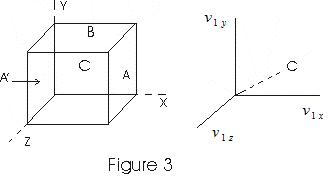# Kinetic Theory Of Gases

## 3. Moleular nature of matter

• We know that molecules which are made up of one or more atoms constitute matter.
• In solids these atoms and molecules are rigidly fixed and space between then is very less of the order of few angestrem and hance they can not move.
• In liquids these atoms and molecules can more enabeling liquids to flow.
• In gases atoms are free to travell without colliding for large distances such that if gases were not enclosed in an enclosure they would disappear.

## 4. Dalton's law of partial pressures :

• Consider a mixture of non-interacting ideal gases with n1 moles of gas 1,n2 of gas 2 and so on
• Gases are enclosed in an encloser with volume V, temperature T and pressure P.
• Equation of state of mixture
PV = (n1+ n2)RT
or     P = n1RT/V + n2RT/V + - - - -
= P1 + P2 + - - --
where,
P1 = n1RT/V
is pressure the gas 1 would exert at same V and T if no other gases were present in the enclosure. This is know as law of partial pressure of the gases.
• The total pressure of mixture of ideal gases is sum of partial pressures of individual gases of which mixture is made of.

## 5. Kinetic Theory of an ideal gas

Following are the fundamental assumptions of kinetic theory of gases.
• Gas is composed of large number of tiny invisible particles know as molecules
• These molecules are always in state of motion with varying velocities in all possible directions.
• Molecules traverse straight line path between any two collisions
• Size of molecule is infinitely small compared to the average distance traverse by the molecules between any two consecutive collisions.
• The time of collision is negligible as compared with the time taken to traverse the path.
• Molecules exert force on each other except when they collide and all of their molecular energy is kinetic.
• Intermolecular distance in gas is much larger than that of solids and liquids and the molecules of gas are free to move in entire space free to them.

## 6. Pressure of gas

• Consider a cubical vessel with perfectly elastic walls containing large number of molecules say N let l be the dimension of each side of the cubical vessel.
• v1x , v1y, v1z be the x, y, and z componenet of a molecule with velocity v.
• Consider the motion of molecule in the direction perpandicular to the face of cubical vessel.
• Molecule strikes the face A with a velocity v1x and rebounds with the same velocity in the backward direction as the collisions are perfectly elastic.• If m is the mass of molecule, the change in momentum during collision is
mv1x - (-mv1x) = 2 mv1x          (1)
• The distance travelled parallel to x-axis an is between A to A´ and when molecule rebounds from A´and travel towards A is 2L
• Time taken by molecule to go to face A´ and then comeback to A is
Δt = 2l/v1x
• Number of impacts of this molecule with A in unit time is
n = I/Δt = v1x/ 2l               (2)
Rate of change of momentum is
ΔF = ΔP/Δt
=nΔP
from (1) and (2)
ΔF = mv1x2 / l
this is the force exerted on wall A due to this movecule.
• Force on wall A due to all other molecules
F = Σmv1x2/L           (3)
• As all directions are equivalent
Σv1x2=Σv1y2=Σv1z2
Σv1x2= 1/3Σ((v1x)2 + (v1y)2 +( v1z)2 )
= 1/3 Σv12
Thus     F = (m/3L) Σv12
• N is total no. of molecules in the container so
F = (mN/3L) (Σ(v1)2/N)
• Pressure is force per unit area so
P = F/L2
=(M/3L3)(Σ(v1)2/N)

where ,M is the total mass of the gas and if ρ is the density of gas then
P=ρΣ(v1)2/3N
since Σ(v1)2/N is the average of squared speeds and is written as vmq2 known as mean square speed
Thus, vrms=√(Σ(v1)2/N) is known as roon mean squared speed rms-speed and vmq2 = (vrms)2
• Pressure thus becomes
P = (1/3)ρvmq2                     (4)
or     PV = (1/3) Nmvmq2                     (5)
from equation (4) rms speed is given as
vrms = √(3P/ρ)
= √(3PV/M)
(6)

link to this page by copying the following text